Subject:
Ratios and Proportions
Material Type:
Lesson Plan
Level:
Middle School
7
Provider:
Pearson
Tags:
• Money
• Percentages
Language:
English
Media Formats:
Text/HTML

# Understanding Percent Change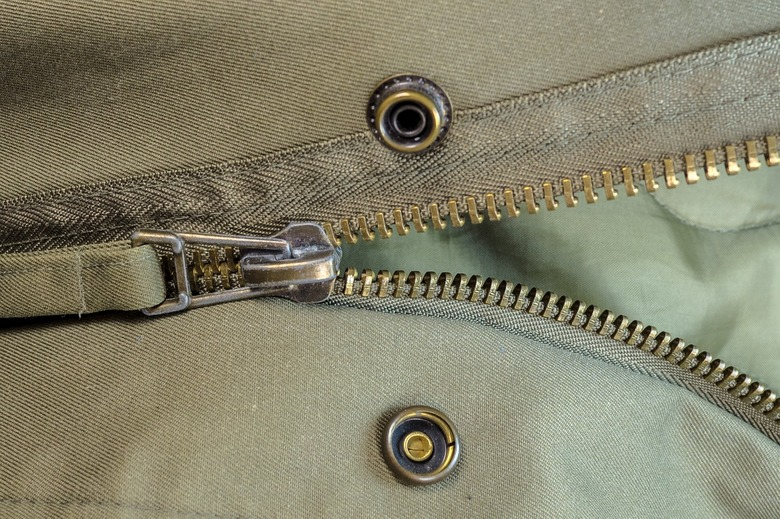## Overview

In Part l of this two-part lesson, students use an interactive to place percent increase and percent decrease signs between monetary amounts to indicate the correct increase or decrease between the amounts of money. They must also place the correct decimal multiplier  between the two amounts to show what decimal to multiply the original amount by to get the final amount.

# Key Concepts

Students apply understanding of percent change situations to systematize and generalize patterns in relating two amounts by multiplication.

# Goals and Learning Objectives

• Identify the percent increase or percent decrease between two amounts.
• Identify the decimal multiplier that when multiplied by the original amount results in the final amount.
• Reason abstractly and quantitatively.

# Lesson Guide

• Have pairs of students discuss and reach an agreement about which two dollar amounts have a 25% increase between them.
• Then have students reflect on their discussion in light of Mathematical Practice 2: Reason abstractly and quantitatively. Ask students to discuss the merits of this practice in this context.
• As a whole class, have students share their ideas about what abstractly and quantitatively each mean in this context.

# Mathematics

This two-part Putting It Together lesson presents opportunities for students to use Mathematical Practice 2: Reason abstractly and quantitatively to help them think about percent change problems.

Have students share ideas about what abstractly and quantitatively mean in the context of identifying an increase of 25% between two dollar amounts.

Facilitate this discussion to bring out ideas related to contextualizing the mathematics—making sense of values and operations in terms of the meaning they have in a situation (i.e., thinking quantitatively)—and to bring out ideas related to decontextualizing the mathematics—making sense of values and operations structurally or formally, as symbols that can be manipulated and simplified (i.e., thinking abstractly).

Highlight the point that alternating between both ways of thinking within the same problem context is powerful.

ELL: Target and model key language and vocabulary. Specifically, focus on the terms abstractly, quantitatively, contextualize, anddecontextualize. As you’re discussing the key points, write the words on the board or on large sheets of paper and explain or demonstrate what the words mean. Since these are important points that students will use throughout the unit, consider writing them on large poster board that students can use as a reference. Have students record new terms, definitions, and examples in their Notebook.

# Reason Abstractly and Quantitatively

In this activity, there are four amounts of money and a set of arrows with different percent increases or percent decreases. Your job will be to place the arrows with the correct increase and decrease between each stack of money.

Discuss the following with your classmates.

• Can you find two dollar amounts that have a 25% increase between them?
• Do you think you'll be able to find the correct increase and decrease values for each arrow on the money board?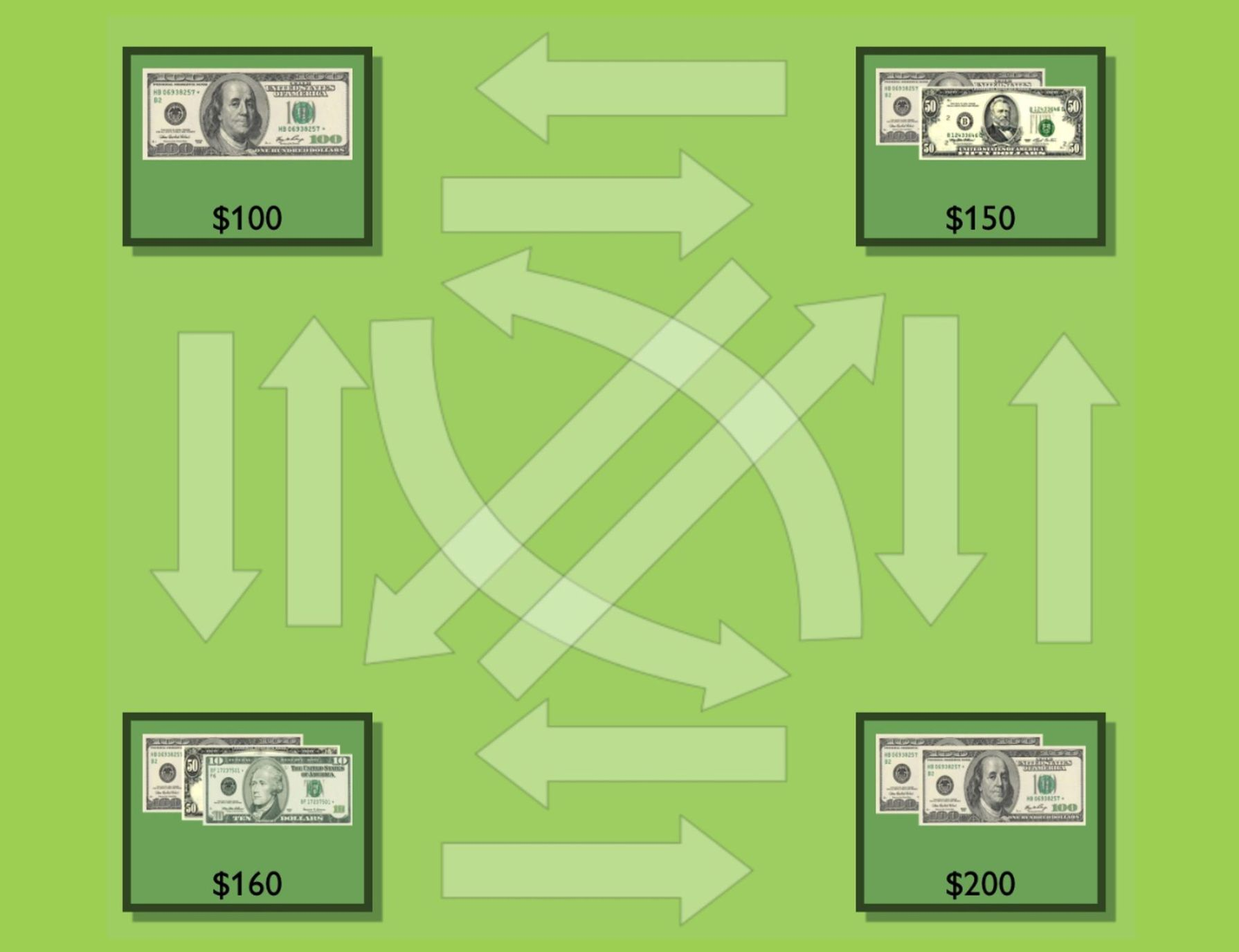# Lesson Guide

Discuss the Math Mission. Students will relate percent increase and decrease to decimal and fraction multipliers.

## Opening

Relate percent increase and decrease to decimal and fraction multipliers.

# Lesson Guide

Have students work in pairs with the Money Card Sort interactive, taking turns placing percent change between two dollar amounts. With each turn, the student making the placement explains his or her thinking, and the partner challenges the placement if he or she disagrees. Have partners work on the presentation together, too.

SWD: Composing mathematical explanations can be challenging for students with disabilities. Use the following supports for struggling students:

• Provide students with a word bank of terms that will help them to structure their response in this task (include the terms increase and decrease).
• Provide students with sentence starters.

# Mathematical Practices

Mathematical Practice 7: Look for and make use of structure.

Make sure that students are following a system of steps and organization in using percents, decimals, and fractions interchangeably.

Mathematical Practice 2: Reason abstractly and quantitatively.

Pay attention to how students are using real-world contexts to give meaning to the monetary values and the percent change amounts and the decimal multipliers. Also notice how students are reasoning abstractly about the values, including the mathematical structure of percent change situations explored in previous lessons.

Mathematical Practice 3: Construct viable arguments and critique the reasoning of others.

Students must make the case to their partner for each percent change and decimal multiplier placement and must critique one another’s reasoning by challenging placements they disagree with during each turn.

# Interventions

Student struggles to get started.

• How can you figure out the percent difference between these two dollar amounts?
• Here are two ways of tackling this task: (1) work out the percent difference between two dollar amounts; (2) take a percent change arrow and use guess and check to try it out between different dollar amounts.
• Find the percent change arrow that says “down by 25%.” If $200 decreases by 25% what would be its new value? Does your answer match any of the dollar amounts on the money mat? [common error] Student makes mistakes in reasoning about percent change in both directions between two dollar amounts. • The price of a blouse is$20. It increases by $\frac{1}{2}$. What is the new price? [$30] • The price of the blouse now decreases by $\frac{1}{2}$. What is the final price? [$15]
• Now apply this thinking to percents. What happens if the $20 blouse increases by 50%? What happens now when this new price decreases by 50%? What percent should the new price have decreased by to get it back to$20? 33 1/3% What does this show?

Students are not challenging one another or constructing arguments about arrow placements.

• Tell your partner why you placed this card here. Then make an argument for why this placement is correct or incorrect.

[common error] Student understands the general idea but misplaces an arrow due to a miscalculation.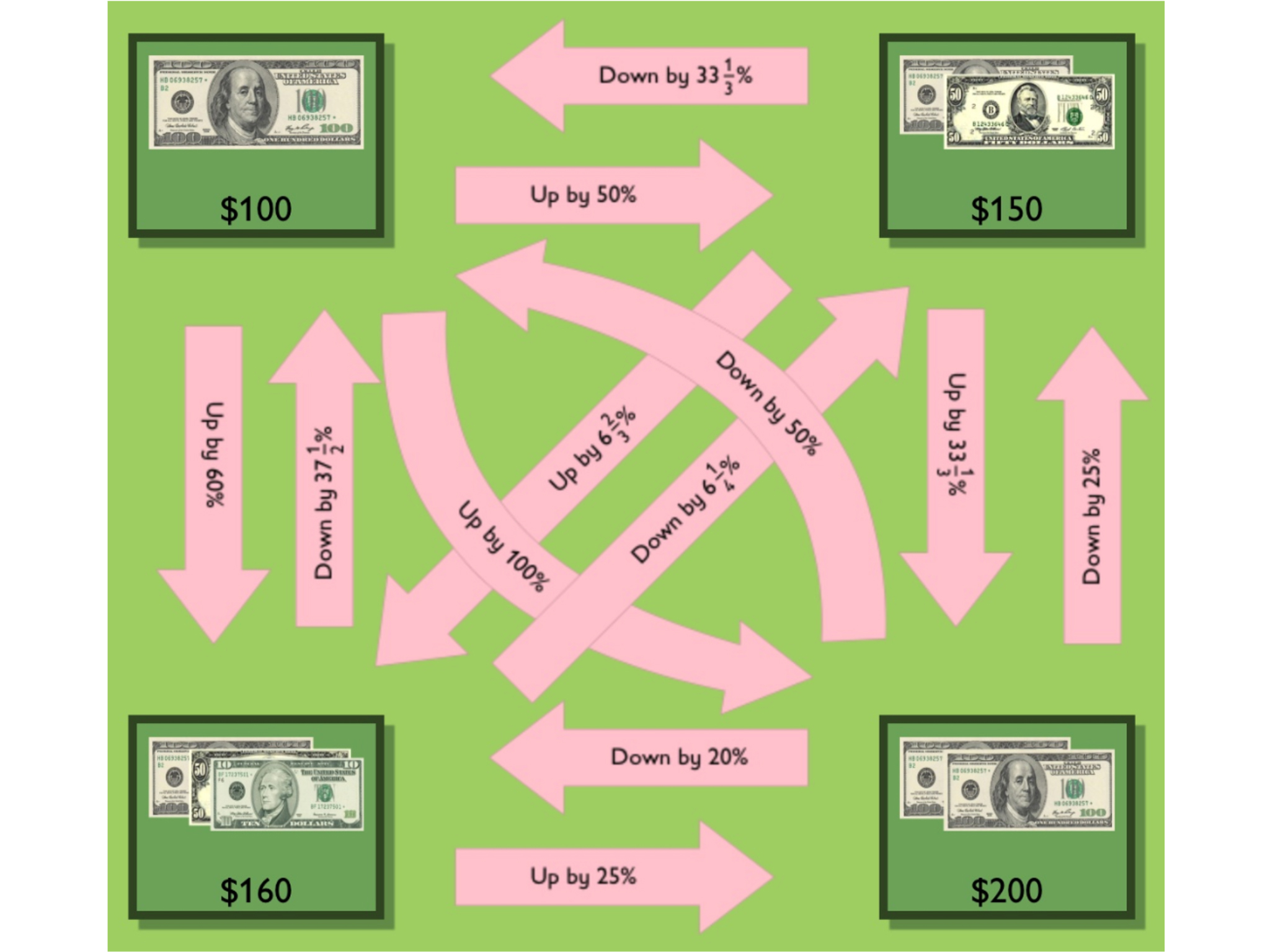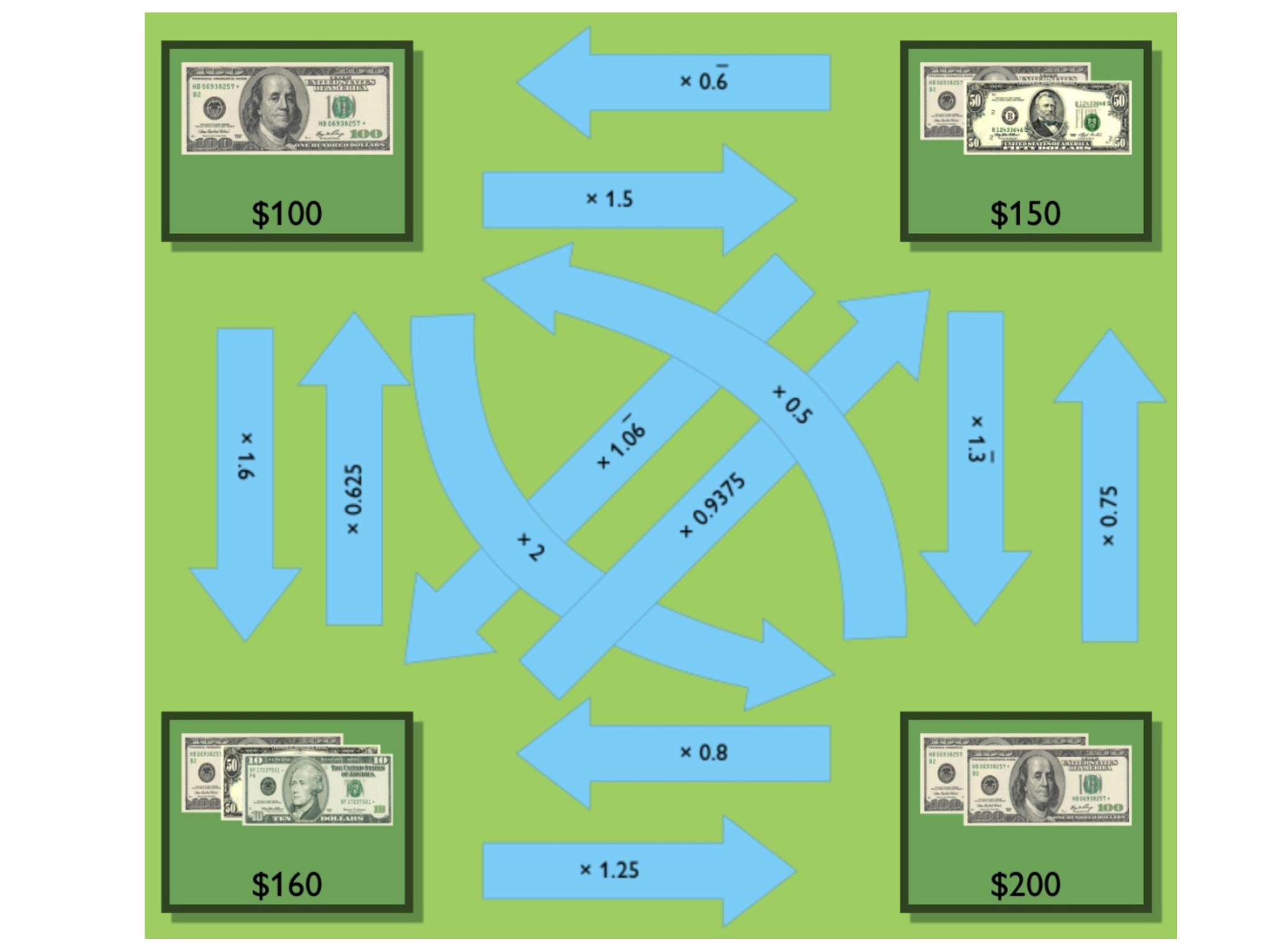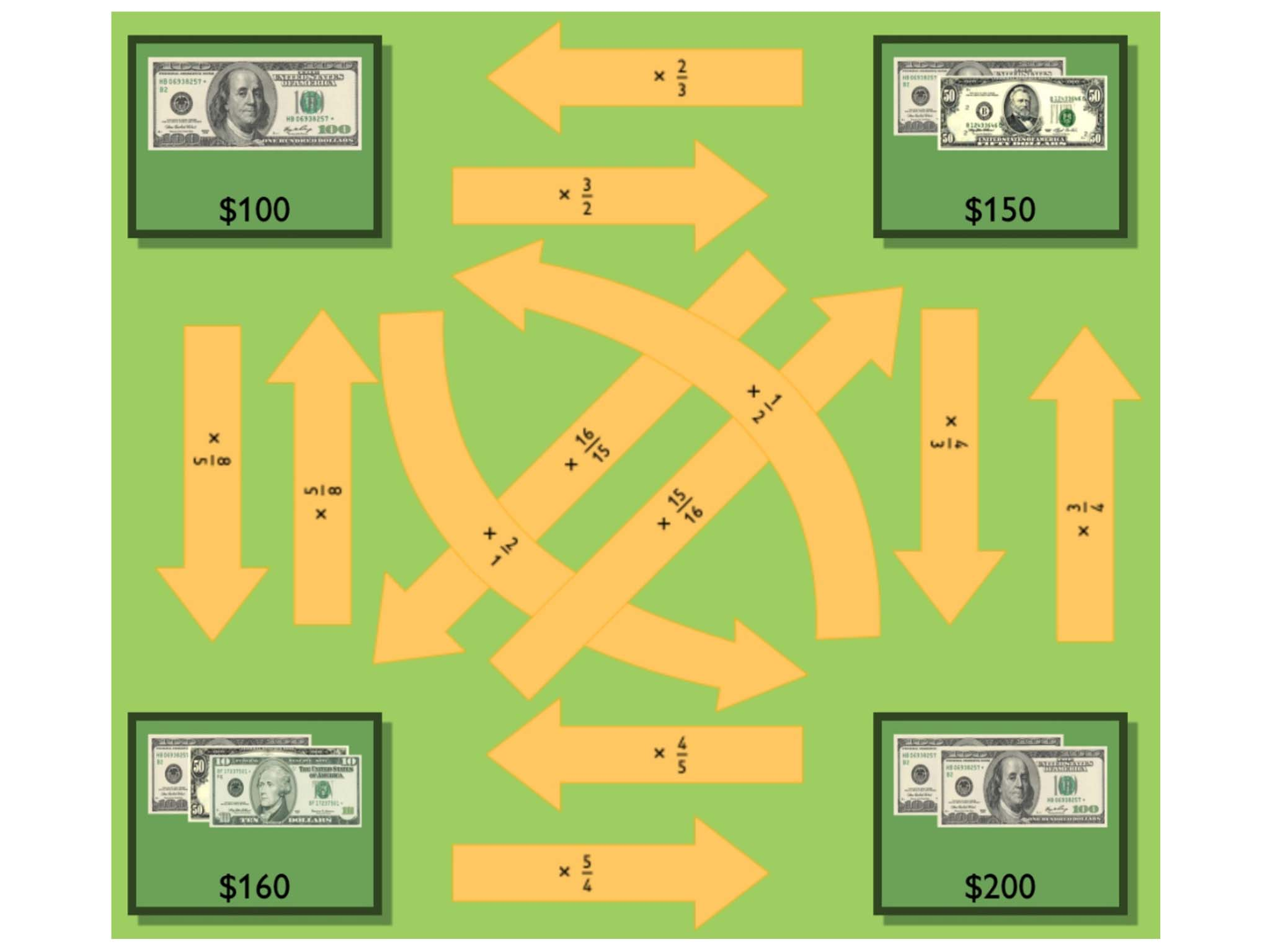# Show Increases and Decreases

Using the Money Card Sort interactive, place the increase and decrease arrows, which contain percent, decimal, and fraction values, between the monetary values to indicate the correct increase or decrease between the amounts of money. The arrows should be placed both horizontally and vertically.

INTERACTIVE: Money Card Sort

# Preparing for Ways of Thinking

Listen and look for students who:

• Recognize early that percent change arrows pointing in different directions between the same two dollar amounts do not have the same percent values.
• Discuss the fact that getting from one amount to another, and in the reverse direction, requires different percent values.
• Have difficulty moving from the percent change cards to the decimal multiplier cards.
• For the Challenge Problem, discuss how to use the blank arrow cards and how to express the percent change or decimal multipliers on them.

# Challenge Problem

• See diagonal arrows.# Prepare a Presentation

Be prepared to show and justify your placement of the arrows.

# Challenge Problem

Find the percent change and decimal multiplier values that lie between the diagonals $150/$160 and $100/$200.

INTERACTIVE: Money Card Sort 2

# Mathematics

As students present their work, bring out multiple ways of explaining or justifying the arrow placements that were hardest for most students.

Ask students to look for generalizations and patterns in each other’s work—for example:

• An increase of, say, $33\frac{1}{3}%$ is equivalent to multiplying by $1.\overline{3}$. Check that students understand that an increase of 5% is equivalent to multiplying by 1.05, not 1.5.
• A decrease of, say, $33\frac{1}{3}%$ is equivalent to multiplying by $\left(1-0.\overline{3}\right)$ or $0.\overline{6}$.
• The “inverse” of an increase by a percent is not a decrease by the same percent.

Help students see that when the decimal multipliers are considered in pairs, a calculator will show that each pair multiplies to give 1, subject to rounding by the calculator.

SWD: Revisit the vocabulary introduced in this lesson. As students present their solutions to the Work Time problems, make note of key words and write them on a chart. Provide plenty of repetition and review of new terminology. Make sure all students have these terms in their Notebook.

ELL: In working with ELLs during the Ways of Thinking section:

• Speak clearly and monitor your speech for slang and idiomatic expressions.
• Use intonation, volume, and pauses to help make the meaning clear.
• Rephrase instead of repeating; paraphrase important concepts and directions.

# Mathematical Practices

Mathematical Practice 2: Reason abstractly and quantitatively.

Look for students who used experiences from previous lessons with real-world percent change problems, as well as their own experiences, to think through the problems in terms of meaningful situations.

### Mathematical Practice 7: Look for and make use of structure.

Point out students who recognize and use the structure of percent change problems examined in previous lessons to make the work of placing decimal multipliers easier and more efficient.

Mathematical Practice 3: Construct viable arguments and critique the reasoning of others.

Identify partners who carry out productive discussions to resolve points of disagreement about arrow placements and who develop arguments to communicate reasoning, resulting in deeper understanding for both partners.

# Ways of Thinking: Make Connections

Take notes about your classmates’ explanations of their placement of the arrows.

## Hint:

• How did you figure out the percent change between those two amounts of money?
• Why did you place the arrow in that position?
• Why aren’t the percent increase and the percent decrease values the same?
• How did you determine which fraction multiplier was the same as that percent change?
• How did you determine which decimal multiplier was the same as that percent change?

# A Possible Summary

When considering a percent change in value between two amounts, the direction of the change matters for the multiplier between the amounts. The starting amount is treated as 1, or “the whole,” and the change amount is proportional to that amount: It is a percent of that whole. The multiplier tells you what portion of the starting amount is added or subtracted (to/from the starting amount) to get the final amount.

SWD: Some students may struggle with the task of writing a summary of the mathematics from the lesson. Possible supports:

• Prior to writing the summary, have students discuss their ideas with a partner or adult and rehearse what they might write.
• Allow students to map out their ideas in outline form or in a concept web.

# Summary of the Math: Percent Increase and Decrease

Write a summary about using percent increase and decrease—as well as decimal and fraction multipliers—to show increases and decreases in quantities.

## Hint:

• Do you explain how to calculate a percent increase and a percent decrease?
• Do you explain how you can represent percent increase and decrease using decimal and fraction multipliers?